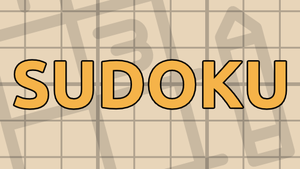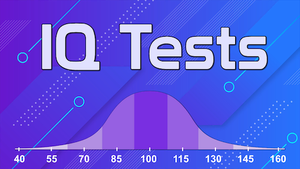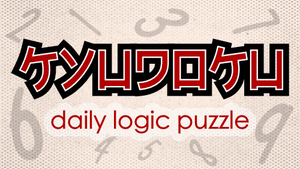• B + E = A
• A + C = D
• C + F = B

• A + G = F
• A + C = B
• F + G = C
• D + F = E

• B + E = F + 2
• C + E = D + 6
• D + G = C + 5
• B + G = D + 5

• B + C = A + 6
• D + G = B + 1
• C + E = F + 7
• E + G = B + 4

• E = C + 6
• G = D + 2
• F = A + 2
• G = B + 4

• G = E + 1
• D = C + 1
• A = B + 6
• C = G + 1
• E = F + 1

• B = 4D
• A = 3C
• 4E = 3B
• G ≠ 5

• 2C = D
• 2A = 3B
• A = 2F
• E ≠ 5

• 3E = C
• G = 2F
• D = 3F
• B > A

• 4A = 3D
• 4B = 2D
• G > B
• 3B = C
• A > E
• G > F

• E + G = D + F
• G ≠ 5
• B + E = D + G
• C + D = A + B
• G ≠ 6
• A ≠ 5
• A ≠ 7

• C + F = E + G
• A + D = C + G
• D + F = B + E
• G ≠ 2
• E + F = C + D

• 2C = B + F
• B > F
• 2A = B + E
• E > A
• 2G = A + B

• 2F = A + D
• 2E = A + C
• 2E = B + D
• 2A = E + F
• B > E

• A + E ≤ 3
• F + G ≤ 8
• B + G = C
• B + E = D

• D + G = C
• B + D = A
• B + F ≤ 3
• E + F ≤ 7
• A + D ≤ 8

• C + D + F = 10
• F + G = 11
• A + C + F = 9
• E + G = 8

• A + F = 10
• B + G = 10
• B + C = 7
• B + F = 9
• A + C + E = 10

• if C < 5 then A > 7
• if A + E ≤ 12 then D ≤ 4
• if A ≤ 4 then E + F ≤ 9
• if G ≥ 4 then C < 5
• if F < 4 then E ≤ 5
• if G ≤ 2 then D ≠ 1
• if C + D ≤ 10 then A + F ≤ 4
• if C ≠ 4 then A ≥ 2
• if G ≥ 1 then F ≤ 2
• if E + F ≤ 11 then A < 3
• if A + G ≤ 7 then B ≠ 6

• if F < 6 then D > 4
• if F ≤ 4 then E + G ≤ 3
• if B < 7 then C + D ≤ 3
• if A ≠ 2 then D < 3
• if C ≤ 6 then B + G ≤ 7
• if E + G ≤ 8 then A ≤ 5
• if D ≤ 1 then B + E ≤ 7
• if G ≠ 4 then C + F ≤ 4
• if D ≤ 4 then E ≠ 6
• if C + D ≤ 5 then F ≤ 6
• if D < 3 then A > 5

• D + E ≤ 3 if and only if F ≤ 2
• E + G ≤ 8 if and only if C < 1
• D + F ≤ 12 if and only if B < 6
• B + D ≤ 9 if and only if C + G ≤ 12
• G ≠ 3 if and only if B < 6
• B + D ≤ 9 if and only if A + F ≤ 12
• D + E ≤ 6 if and only if B ≠ 7
• A > 1 if and only if B > 4
• C ≠ 4 if and only if F ≤ 4
• C ≤ 7 if and only if A > 2
• E < 3 if and only if B ≥ 2
• F < 4 if and only if C < 1

• A + B ≤ 12 if and only if F + G ≤ 8
• A > 2 if and only if C + E ≤ 5
• F ≥ 6 if and only if A ≤ 5
• C ≥ 3 if and only if A + B ≤ 12
• F ≤ 7 if and only if C > 5
• B + D ≤ 7 if and only if G < 6
• A ≠ 1 if and only if B ≠ 2
• D + E ≤ 8 if and only if C + G ≤ 13
• E ≠ 4 if and only if C + G ≤ 7

• C = DF
• F = G + 2
• B + D = A + G

• A = B + E + G
• EG + F = B + C
• C = B + F
• A = AG

• DE = 3C + E + F
• B + D = C + 8
• CE + F = A

• G ≥ 2
• D + F = 6
• C + EF + E + F = AE + AG + BG + G
• A + D ≥ C
• G < 4

• D + 6 = G
• B + E ≠ 8
• B + E = C
• F = A + B
• AB > C

• CD = B
• C + F = B + G
• F > B
• C + F + G = E + 9

### More games##### Self-Referential Quiz

Quizzes where you only need logic to solve them.##### Sudoku

Play the classic japanese logic game.##### IQ Tests